Area as a sum

Chapter 7 Class 12 Integrals
Serial order wise

This Question was also asked in CBSE Maths Board Exam - 2020 (Question 34 - Set 65/5/1)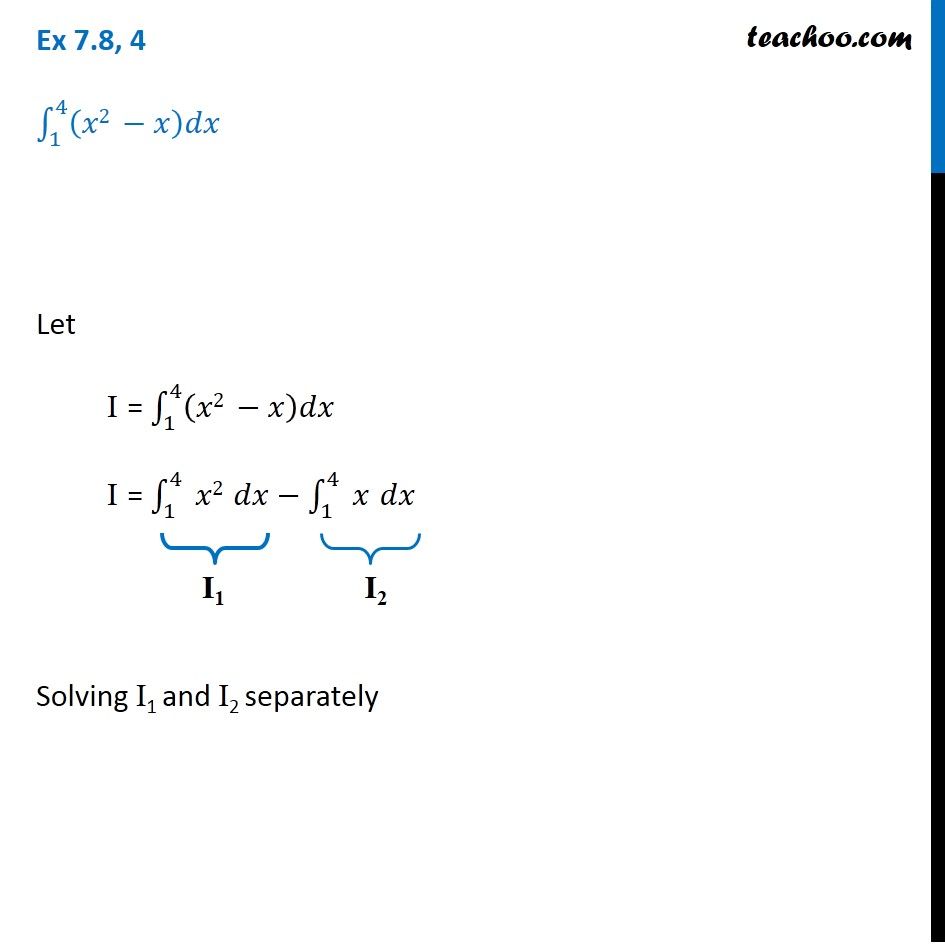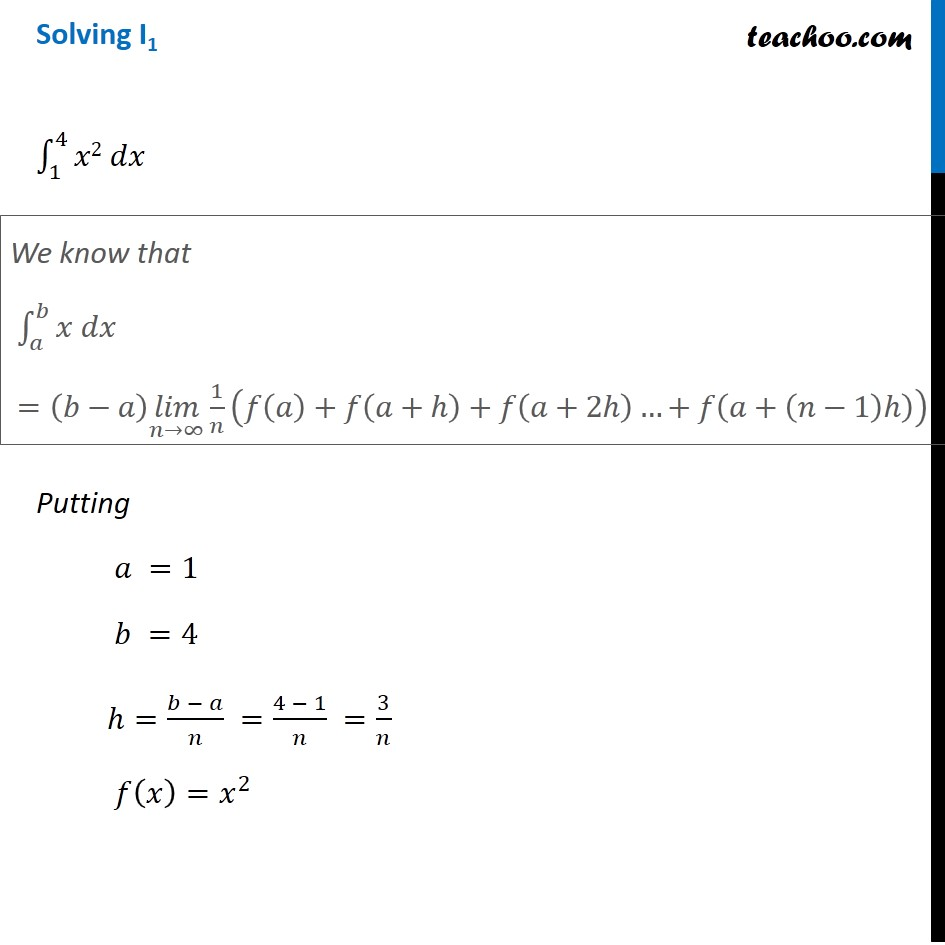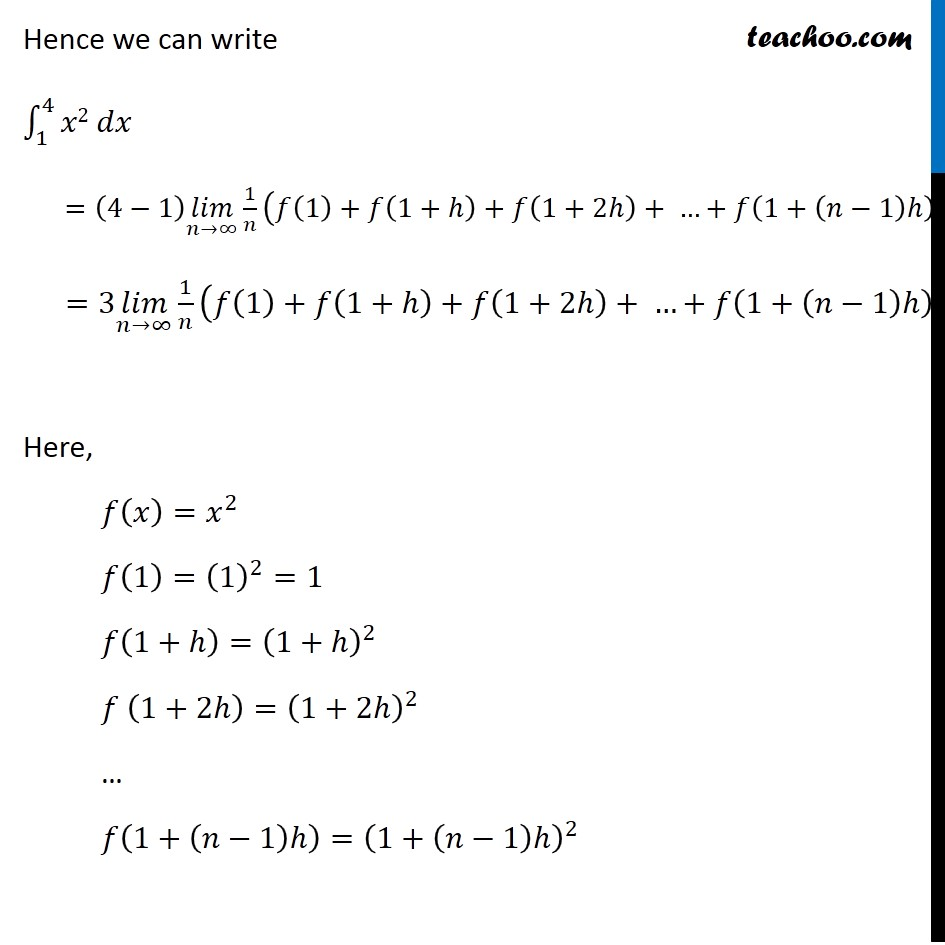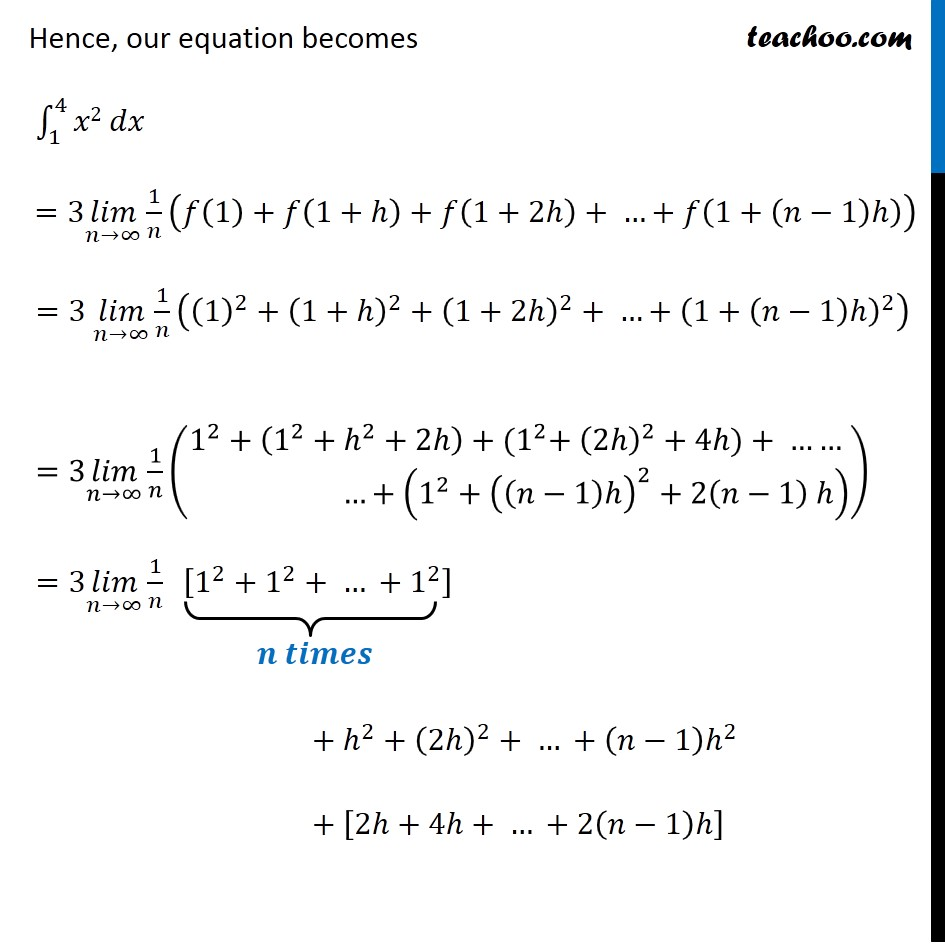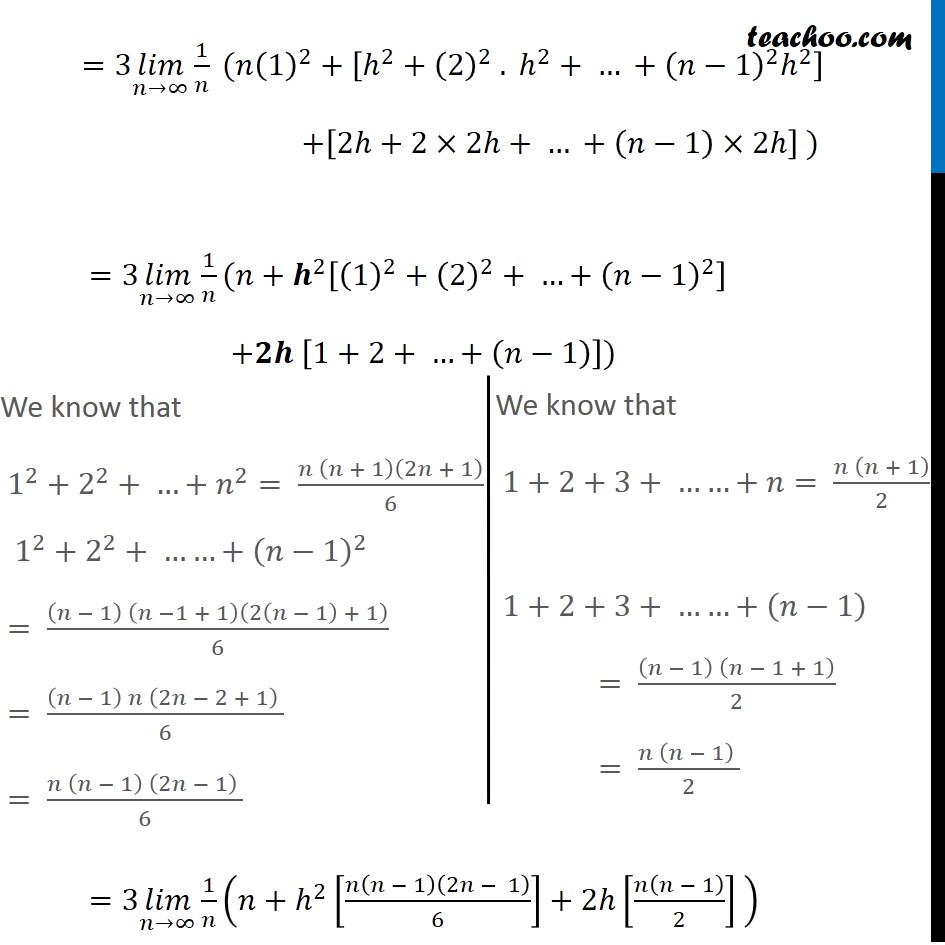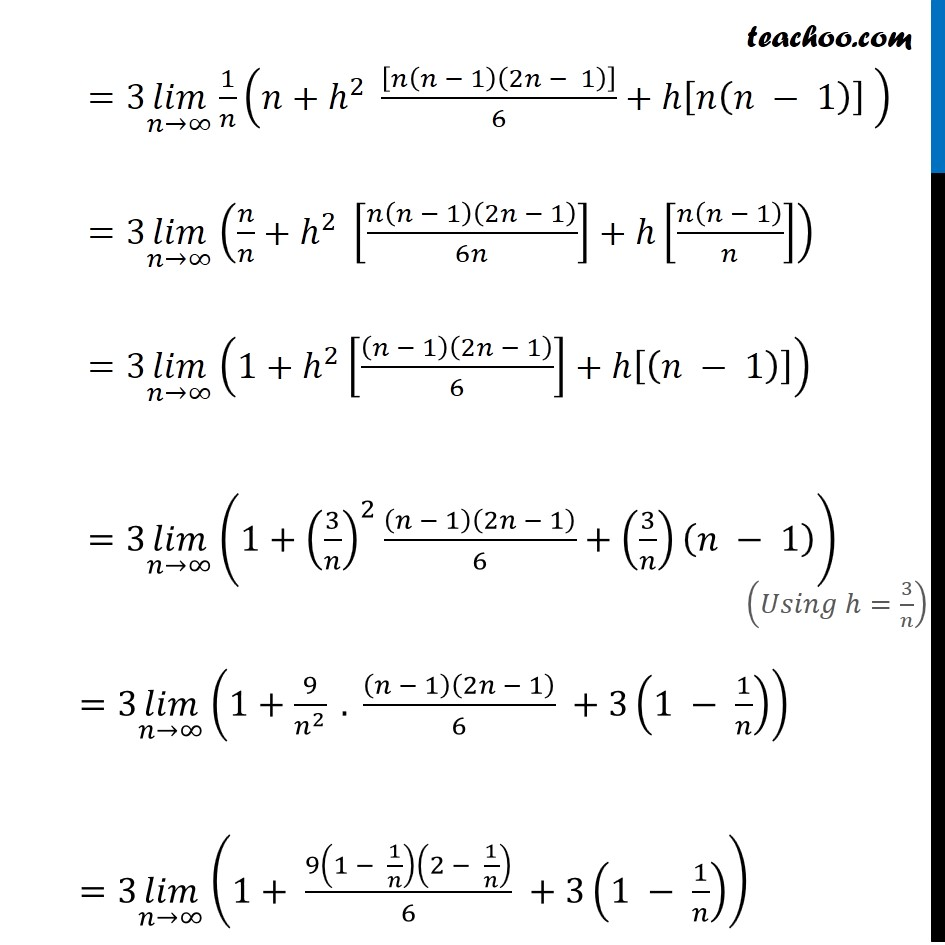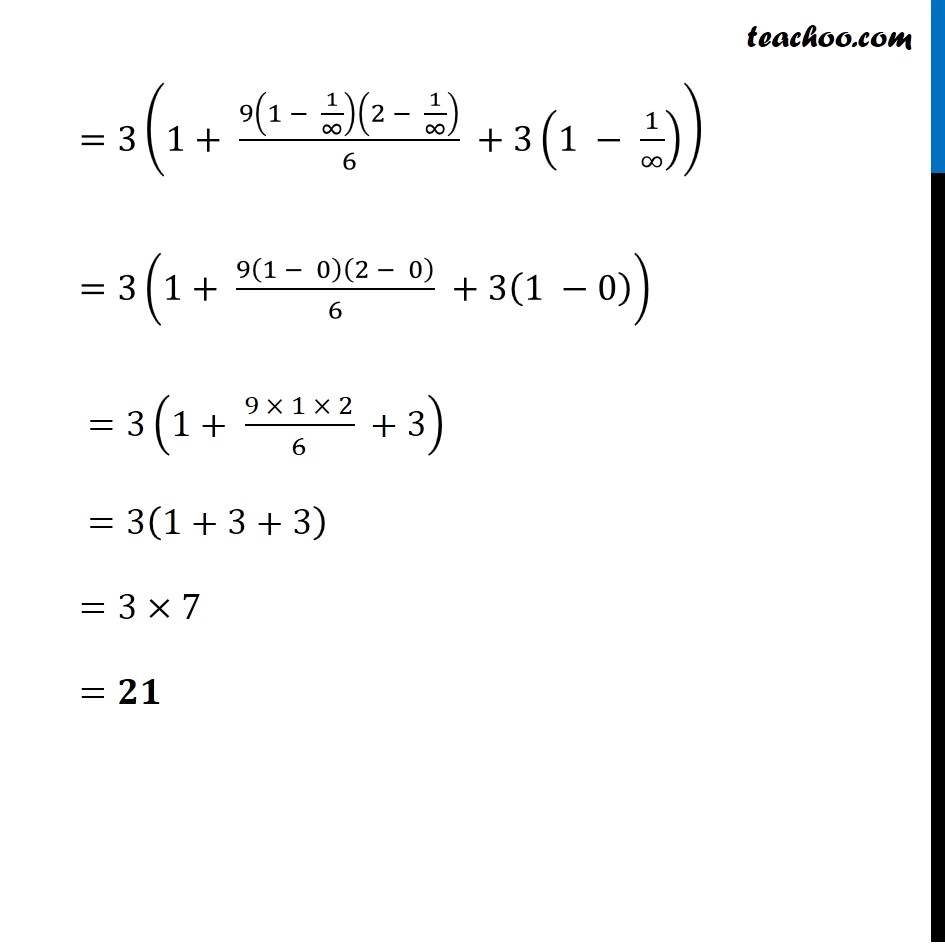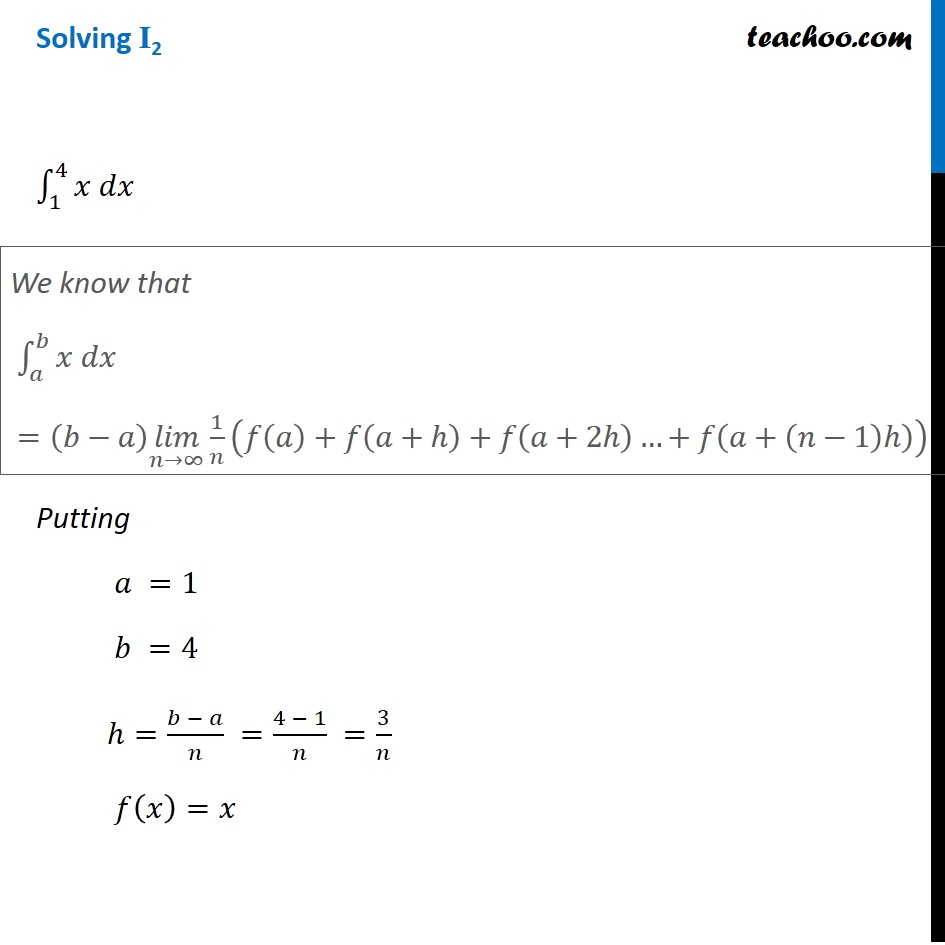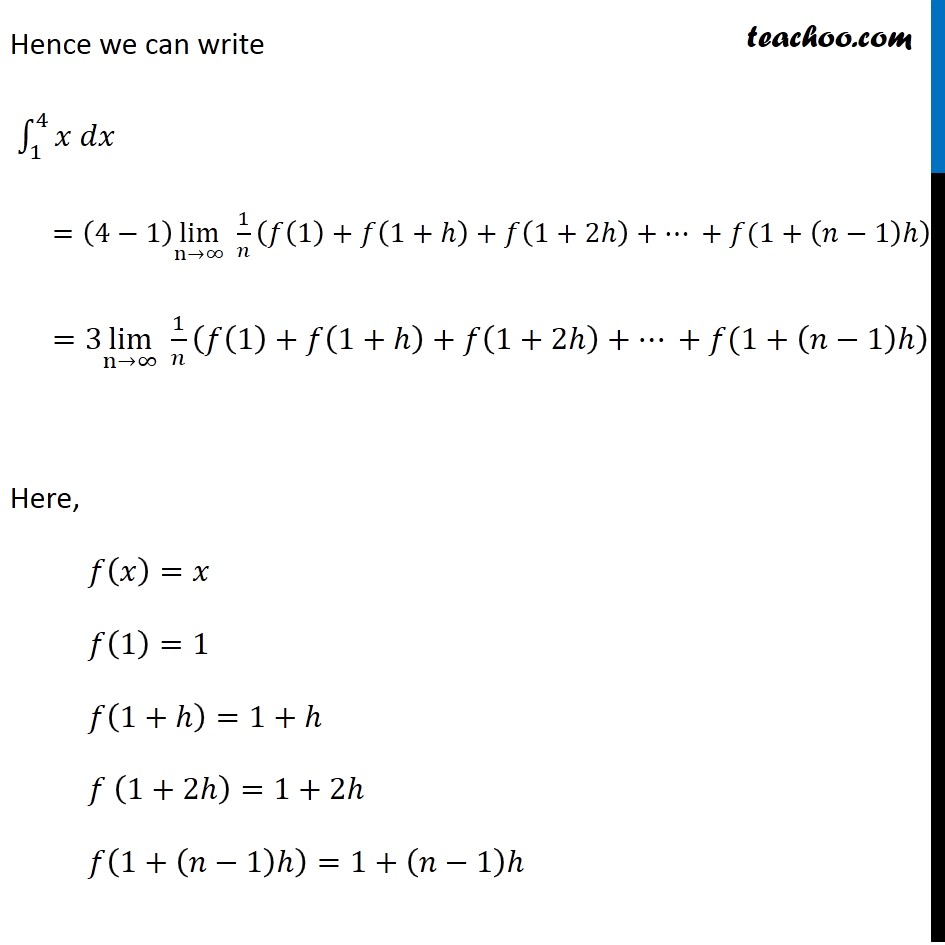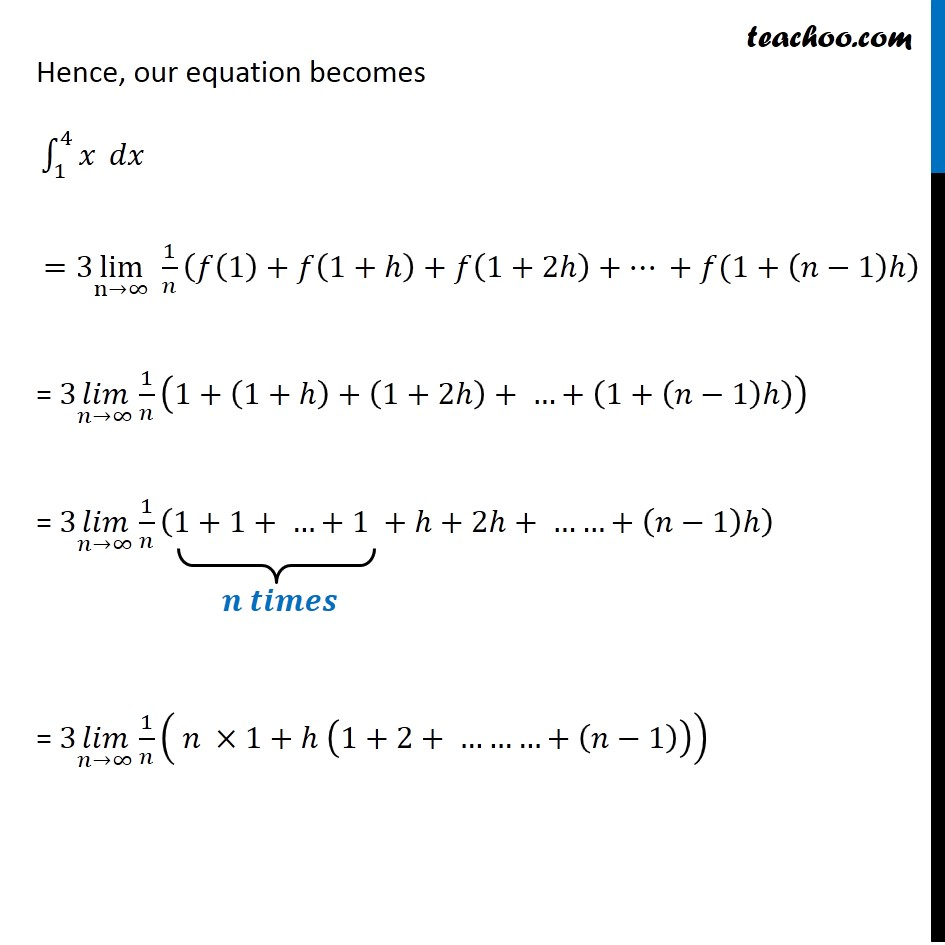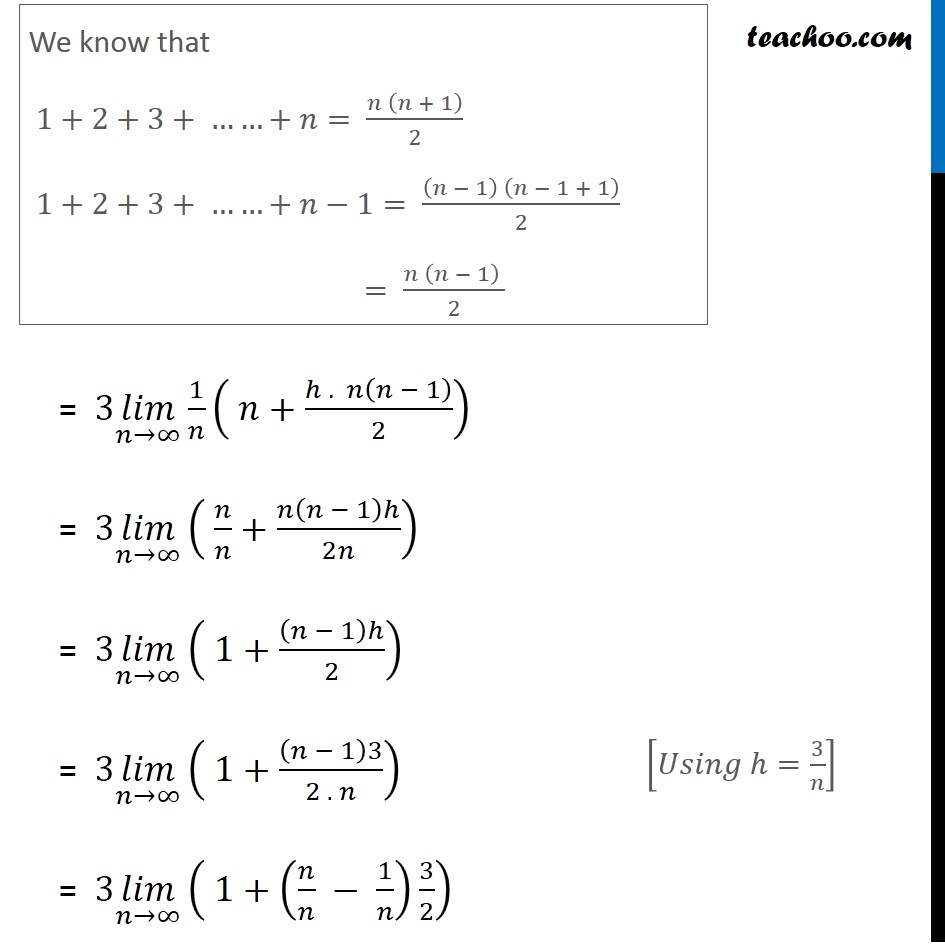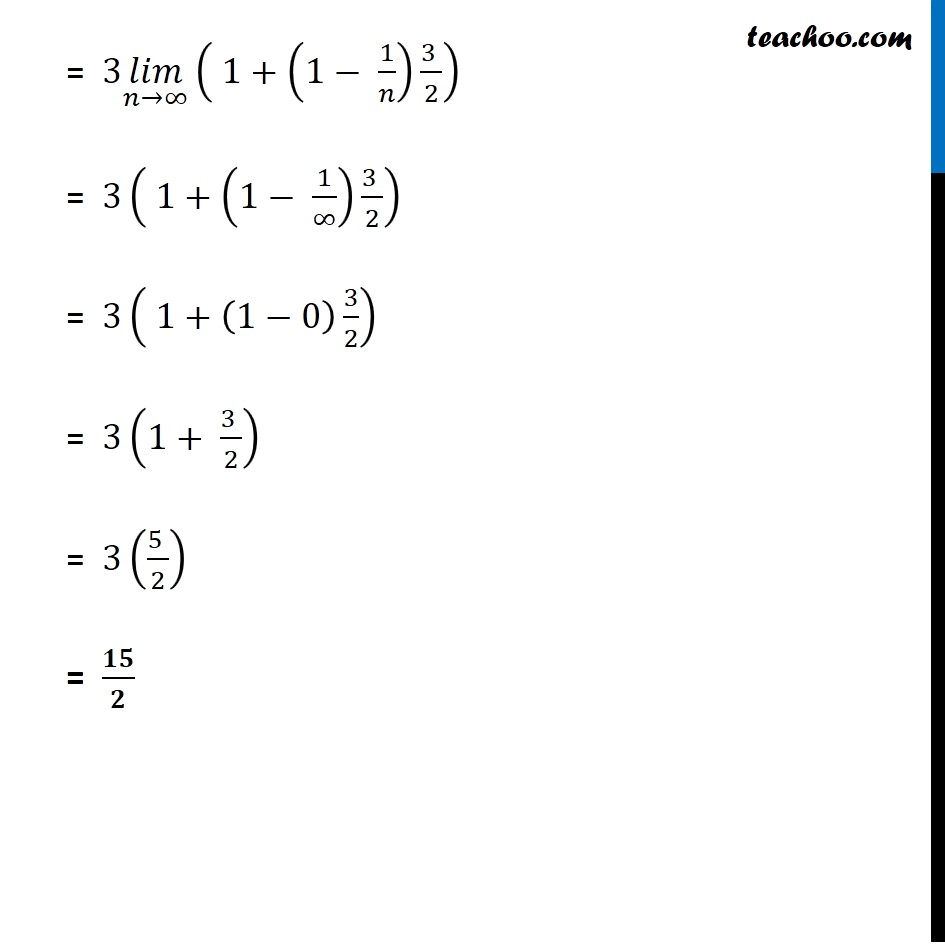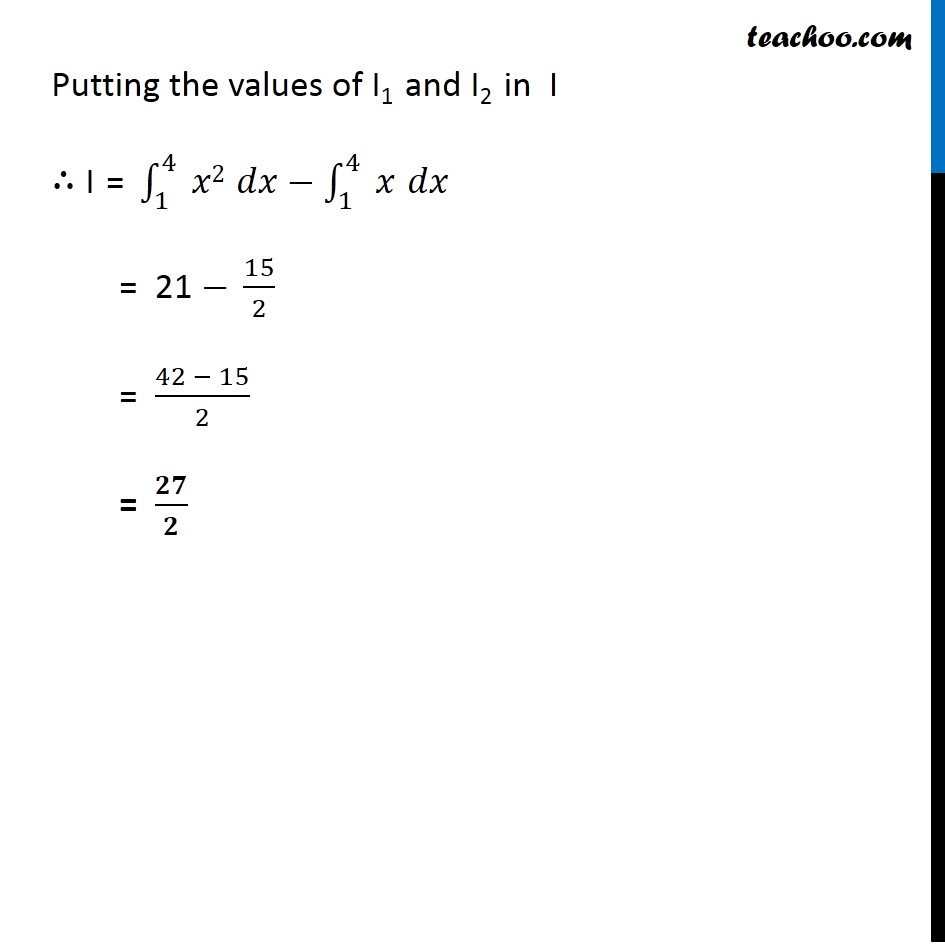Learn in your speed, with individual attention - Teachoo Maths 1-on-1 Class

### Transcript

Question 4 ∫1_1^4▒(𝑥2 −𝑥)𝑑𝑥 Let I = ∫1_1^4▒(𝑥2 −𝑥)𝑑𝑥 I = ∫1_1^4▒〖 𝑥2 𝑑𝑥〗−∫1_1^4▒〖 𝑥 𝑑𝑥〗 Solving I1 and I2 separately Solving I1 ∫1_1^4▒〖𝑥2 𝑑𝑥〗 Putting 𝑎 =1 𝑏 =4 ℎ=(𝑏 − 𝑎)/𝑛 =(4 − 1)/𝑛 =3/𝑛 𝑓(𝑥)=𝑥^2 We know that ∫1_𝑎^𝑏▒〖𝑥 𝑑𝑥〗 =(𝑏−𝑎) (𝑙𝑖𝑚)┬(𝑛→∞) 1/𝑛 (𝑓(𝑎)+𝑓(𝑎+ℎ)+𝑓(𝑎+2ℎ)…+𝑓(𝑎+(𝑛−1)ℎ)) Hence we can write ∫1_1^4▒〖𝑥2 𝑑𝑥〗 =(4−1) (𝑙𝑖𝑚)┬(𝑛→∞) 1/𝑛 (𝑓(1)+𝑓(1+ℎ)+𝑓(1+2ℎ)+ …+𝑓(1+(𝑛−1)ℎ)) =3 (𝑙𝑖𝑚)┬(𝑛→∞) 1/𝑛 (𝑓(1)+𝑓(1+ℎ)+𝑓(1+2ℎ)+ …+𝑓(1+(𝑛−1)ℎ)) Here, 𝑓(𝑥)=𝑥^2 𝑓(1)=(1)^2=1 𝑓(1+ℎ)=(1+ℎ)^2 𝑓 (1+2ℎ)=(1+2ℎ)^2 … 𝑓(1+(𝑛−1)ℎ)=(1+(𝑛−1)ℎ)^2 Hence, our equation becomes ∫1_1^4▒〖𝑥2 𝑑𝑥〗 " " =3 (𝑙𝑖𝑚)┬(𝑛→∞) 1/𝑛 (𝑓(1)+𝑓(1+ℎ)+𝑓(1+2ℎ)+ …+𝑓(1+(𝑛−1)ℎ)) =3 (𝑙𝑖𝑚)┬(𝑛→∞) 1/𝑛 ((1)^2+(1+ℎ)^2+(1+2ℎ)^2+ …+(1+(𝑛−1)ℎ)^2 ) =3 (𝑙𝑖𝑚)┬(𝑛→∞) 1/𝑛 (█(1^2+(1^2+ℎ^2+2ℎ)+〖(1〗^2+ (2ℎ)^2+4ℎ)+ …… @ …+(1^2+((𝑛−1)ℎ)^2+2(𝑛−1) ℎ) )) =3 (𝑙𝑖𝑚)┬(𝑛→∞) 1/𝑛 [1^2+1^2+ … +1^2 ] + ℎ^2+(2ℎ)^2+ … +(𝑛−1)ℎ^2 + [2ℎ+4ℎ+ … +2(𝑛−1)ℎ] =3 (𝑙𝑖𝑚)┬(𝑛→∞) 1/𝑛 (〖𝑛(1)〗^2+[ℎ^2+(2)^2 . ℎ^2+ … +(𝑛−1)^2 ℎ^2 ] +[2ℎ+2×2ℎ+ … +(𝑛−1)×2ℎ] ) =3 (𝑙𝑖𝑚)┬(𝑛→∞) 1/𝑛(𝑛+𝒉^2 [(1)^2+(2)^2+ …+(𝑛−1)^2 ] +𝟐𝒉 [1+2+ …+(𝑛−1)]) =3 (𝑙𝑖𝑚)┬(𝑛→∞) 1/𝑛 (𝑛+ℎ^2 [𝑛(𝑛 − 1)(2𝑛 − 1)/6]+2ℎ[𝑛(𝑛 − 1)/2] ) We know that 1^2+2^2+ …+𝑛^2= (𝑛 (𝑛 + 1)(2𝑛 + 1))/6 1^2+2^2+ ……+(𝑛−1)^2 = ((𝑛 − 1) (𝑛 −1 + 1)(2(𝑛 − 1) + 1))/6 = ((𝑛 − 1) 𝑛 (2𝑛 − 2 + 1) )/6 = (𝑛 (𝑛 − 1) (2𝑛 − 1) )/6 We know that 1+2+3+ ……+𝑛= (𝑛 (𝑛 + 1))/2 1+2+3+ ……+(𝑛−1) = ((𝑛 − 1) (𝑛 − 1 + 1))/2 = (𝑛 (𝑛 − 1) )/2 =3 (𝑙𝑖𝑚)┬(𝑛→∞) 1/𝑛 (𝑛+ℎ^2 [𝑛(𝑛 − 1)(2𝑛 − 1)]/6+ℎ[𝑛(𝑛 − 1)] ) =3 (𝑙𝑖𝑚)┬(𝑛→∞) (𝑛/𝑛+ℎ^2 [𝑛(𝑛 − 1)(2𝑛 − 1)/6𝑛]+ℎ[𝑛(𝑛 − 1)/𝑛]) =3 (𝑙𝑖𝑚)┬(𝑛→∞) (1+ℎ^2 [(𝑛 − 1)(2𝑛 − 1)/6]+ℎ[(𝑛 − 1)]) =3 (𝑙𝑖𝑚)┬(𝑛→∞) (1+(3/𝑛)^2 (𝑛 − 1)(2𝑛 − 1)/6+(3/𝑛)(𝑛 − 1)) =3 (𝑙𝑖𝑚)┬(𝑛→∞) (1+9/𝑛^2 . (𝑛 − 1)(2𝑛 − 1)/6 +3(1 − 1/𝑛)) =3 (𝑙𝑖𝑚)┬(𝑛→∞) (1+ 9(1 − 1/𝑛)(2 − 1/𝑛)/6 +3(1 − 1/𝑛)) =3(1+ 9(1 − 1/∞)(2 − 1/∞)/6 +3(1 − 1/∞)) =3(1+ 9(1 − 0)(2 − 0)/6 +3(1 −0)) =3(1+ (9 × 1 × 2)/6 +3) =3(1+3+3) =3×7 =𝟐𝟏 Solving I2 ∫1_1^4▒〖𝑥 𝑑𝑥〗 Putting 𝑎 =1 𝑏 =4 ℎ=(𝑏 − 𝑎)/𝑛 =(4 − 1)/𝑛 =3/𝑛 𝑓(𝑥)=𝑥 We know that ∫1_𝑎^𝑏▒〖𝑥 𝑑𝑥〗 =(𝑏−𝑎) (𝑙𝑖𝑚)┬(𝑛→∞) 1/𝑛 (𝑓(𝑎)+𝑓(𝑎+ℎ)+𝑓(𝑎+2ℎ)…+𝑓(𝑎+(𝑛−1)ℎ)) Hence we can write ∫1_1^4▒〖𝑥 𝑑𝑥〗 =(4−1) lim┬(n→∞) 1/𝑛 (𝑓(1)+𝑓(1+ℎ)+𝑓(1+2ℎ)+… +𝑓(1+(𝑛−1)ℎ) =3 lim┬(n→∞) 1/𝑛 (𝑓(1)+𝑓(1+ℎ)+𝑓(1+2ℎ)+… +𝑓(1+(𝑛−1)ℎ) Here, 𝑓(𝑥)=𝑥 𝑓(1)=1 𝑓(1+ℎ)=1+ℎ 𝑓 (1+2ℎ)=1+2ℎ 𝑓(1+(𝑛−1)ℎ)=1+(𝑛−1)ℎ Hence, our equation becomes ∫_1^4▒𝑥 𝑑𝑥 =3 lim┬(n→∞) 1/𝑛 (𝑓(1)+𝑓(1+ℎ)+𝑓(1+2ℎ)+… +𝑓(1+(𝑛−1)ℎ) = 3 (𝑙𝑖𝑚)┬(𝑛→∞) 1/𝑛 (1+(1+ℎ)+(1+2ℎ)+ …+(1+(𝑛−1)ℎ)) = 3 (𝑙𝑖𝑚)┬(𝑛→∞) 1/𝑛 (1+1+ …+1 +ℎ+2ℎ+ ……+(𝑛−1)ℎ) = 3 (𝑙𝑖𝑚)┬(𝑛→∞) 1/𝑛 ( 𝑛\ ×1+ℎ (1+2+ ………+(𝑛−1))) We know that 1+2+3+ ……+𝑛= (𝑛 (𝑛 + 1))/2 1+2+3+ ……+𝑛−1= ((𝑛 − 1) (𝑛 − 1 + 1))/2 = (𝑛 (𝑛 − 1) )/2 = 3 (𝑙𝑖𝑚)┬(𝑛→∞) 1/𝑛 ( 𝑛+(ℎ . 𝑛(𝑛 − 1))/2) = 3 (𝑙𝑖𝑚)┬(𝑛→∞) ( 𝑛/𝑛+𝑛(𝑛 − 1)ℎ/2𝑛) = 3 (𝑙𝑖𝑚)┬(𝑛→∞) ( 1+(𝑛 − 1)ℎ/2) = 3 (𝑙𝑖𝑚)┬(𝑛→∞) ( 1+(𝑛 − 1)3/(2 . 𝑛)) = 3 (𝑙𝑖𝑚)┬(𝑛→∞) ( 1+(𝑛/𝑛 − 1/𝑛) 3/2) [𝑈𝑠𝑖𝑛𝑔 ℎ=3/𝑛] = 3 (𝑙𝑖𝑚)┬(𝑛→∞) ( 1+(1− 1/𝑛) (3 )/2) = 3( 1+(1− 1/∞) (3 )/2) = 3( 1+(1−0) 3/2) = 3(1+ (3 )/2) = 3((5 )/2) = 𝟏𝟓/𝟐 Putting the values of I1 and I2 in I ∴ "I = " ∫1_1^4▒〖 𝑥2 𝑑𝑥〗−∫1_1^4▒〖 𝑥 𝑑𝑥〗 = 21 − 15/2 = (42 − 15)/2 = 𝟐𝟕/𝟐